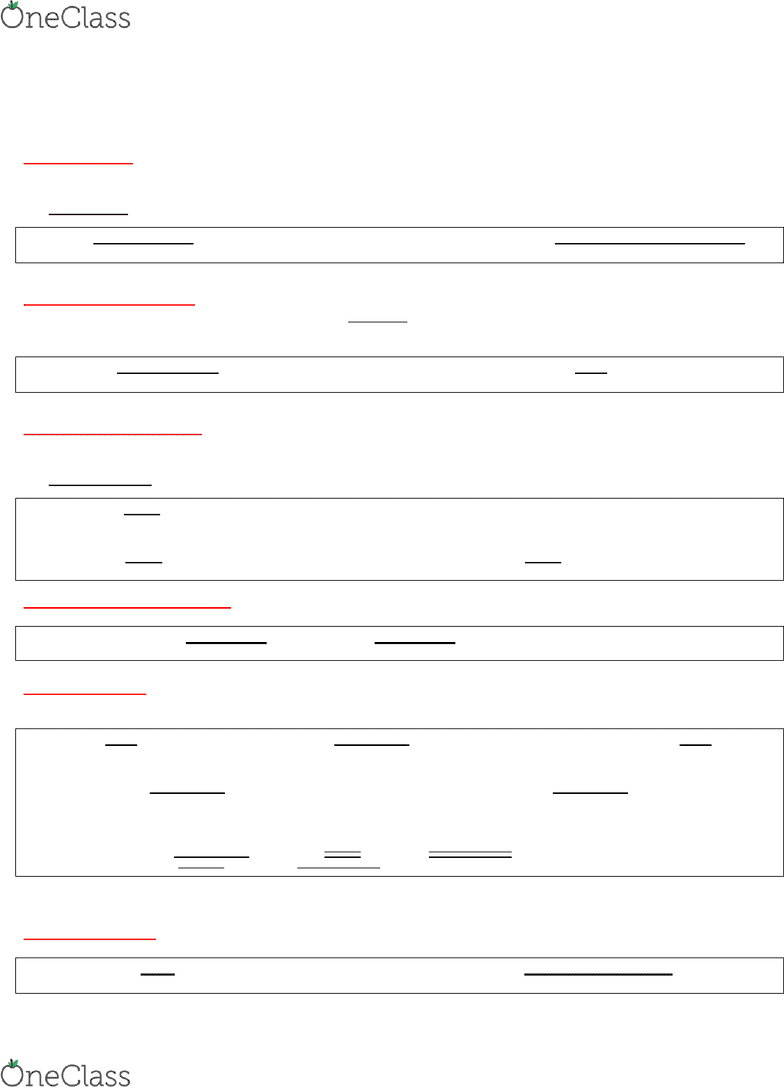Textbook Notes (280,000)
US (110,000)
NU (1,000)
FINA (60)
Chapter 4

# FINA 2201 Chapter Notes - Chapter 4: Trend Analysis, Net Income, Asset Turnover

Department
Finance & Insurance
Course Code
FINA 2201
Professor
Ma Linlin
Chapter
4

This preview shows half of the first page. to view the full 3 pages of the document.FINA 2201 Chapter 4: Analysis of Financial Statements
1
Liquidity ratios: shows the firm’s ability to pay off debts that are maturing within a year.
Asset management ratios: shows how efficiently the firm is using its assets.
Debt management ratios: shows how the firm financed assets as well as the firm’s ability to repay its long-term debt
Profitability ratios: show profitably the firm is operating and utilizing its assets.
Market value ratios: bring in the stock price and of what investors think about the firm & its future prospects.
Liquidity Ratios: show the relationship of a firm’s cash and other current assets to its current liabilities
Will the firm be able to pay off its short-term obligations as they come due?
Indicates extent to which current liabilities are covered by assets expected to be converted to cash in the near future.
Liquid Asset: An asset that can be converted to cash quickly without having to reduce the asset’s price very much.
Current = Current Assets Quick (Acid Test) = Current Assets Inventories
Ratio Current Liabilities (Ratio Current Liabilities
Large values imply that the firm has enough assets to pay its bills on time low probability of insolvency
Debt Management Ratios: A set of ratios that measure how effectively a firm manages its debt.
Will the firm be able to pay off its short-term and long-term obligations as they come due?
How much of every \$ is financed by debt
Debt Ratio = Total Liabilities Times Interest = EBIT
(Leverage) Total Assets Ratio (TIE) Interest Expense
Large Debt Ratio High probability of default Large TIE Low probability of default
Asset Management Ratios: A set of ratios that measure how effectively a firm is managing its assets
Are assets actively and efficiently used to generate returns?
How much of every dollar will generate sales?
Turnover Ratios: how many times the particular asset is “turned over” during the year
Inventory = Sales
Turnover Inventories
Fixed Assets = Sales Total Assets = Sales
Turnover Net fixed assets Turnover Total assets
Days Sales Outstanding (DSO): Indicates the average number of days after making a sale before receiving cash
Days Sales = Receivables = Receivables
Outstanding (DSO) Average sales per day Annual sales/365
Profitability Ratios: Group of ratios that show combined effects of liquidity, asset management & debt on operating
results
How profitable is the firm?
Operating = EBIT Profit = Net Income Basic Earning Power = EBIT
Margin Sales Margin Sales (BEP) Ratio Total Assets
Return On Assets: Net Income Return on Equity = Net income
(ROA) Total assets (ROE) Total Equity
DuPont Equation: ROE = Profit Margin * Total asset turnover * Equity Multiplier
Net Income * Sales * Total Assets
Sales Total Assets Equity
Focuses on profitability (PM), asset utilization (TA TO), and debt utilization (equity multiplier).
shows the relationships among asset management, debt management, and profitability ratios.
Market Value Ratios
how do investors perceive the futures?
Price/Earnings = Price Market/Book = Market price per share
Ratio Earnings per share Ratio Book value per share
How much investors are willing to pay for \$1 How much investors are willing to pay for \$1
of earnings (The higher, the better) of book value equity (the higher, the better)
find more resources at oneclass.com
find more resources at oneclass.com